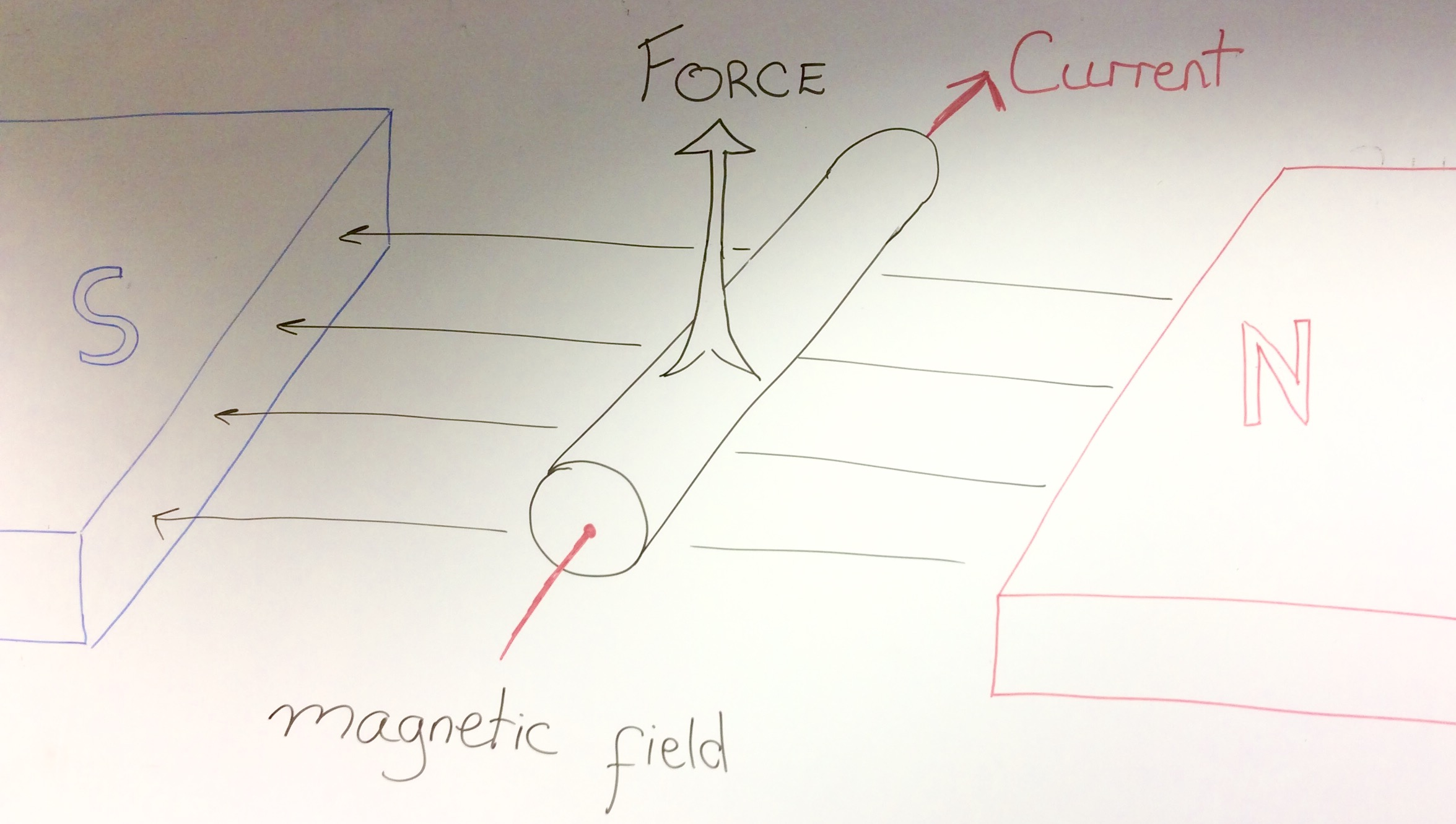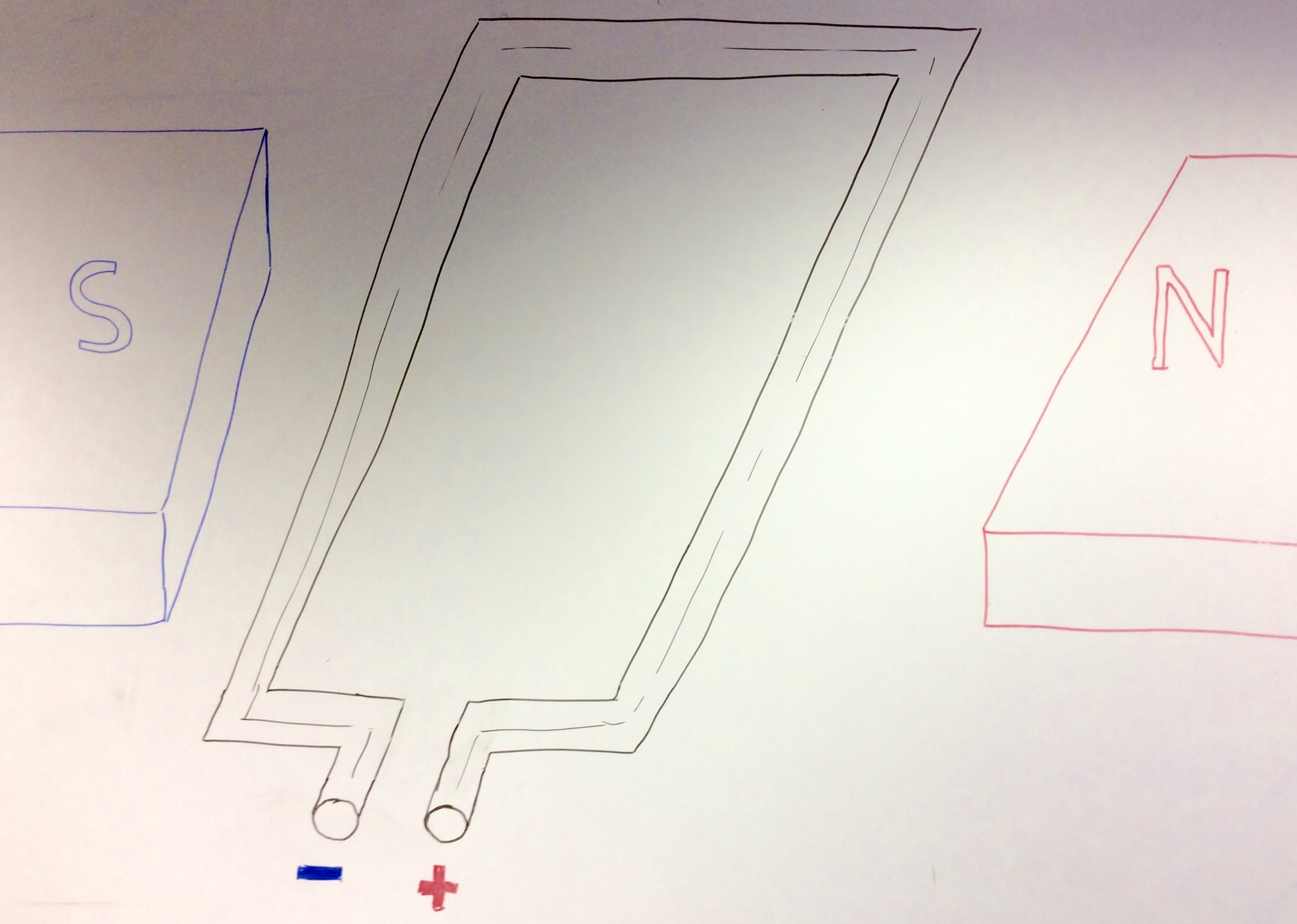# An Introduction to Mechanisms

## 1 Motors

### Learn It

• There are many different forms of energy.
• When you pick up a ball, you give it what is known as Gravitational Potential Energy.
• When you drop it, the Gravitational Potential Energy is converted to Kinetic Energy (movement). In other words, the ball falls.
• Other types of energy are:
1. Electrical Energy
2. Nuclear Energy
3. Thermal Energy (Heat)
4. Light Energy
5. Chemical Energy (Fuel)
6. Elastic Potential Energy.
7. Sound Energy

### Learn It

• Certain devices can convert one form of energy to another.
• A solar panel can convert light energy to electrical energy.
• A wind turbine converts kinetic energy to electrical energy.
• A fire converts chemical energy to light and heat energy.
• In this project you can use a motor. An eclectic motor can convert electrical energy to kinetic energy.

• Click on this link to complete the quiz on the energy conversions that happen in the following systems. Use the web to help you.
1. A Battery
2. An LED
3. A Buzzer
4. A Dynamo
5. A Television.

### Learn It

• An electric motor converts, electrical energy into kinetic energy.
• It achieves this by the use of magnets.
• Look at the diagram below.• When an electric current passes through a wire, that is inside a magnetic filed, the wire experiences a force that will move it.
• In the diagram above:
1. the direction of the magnetic field is from right to left (always N to S).
2. the direction of the current is going into the screen.
3. the force on the wire is upwards.

• If the magnetic field direction is switched, or the direction of current is switched, the force on the wire will also be switched.
1. the direction of the magnetic field.
2. the direction of the current (remember that current flows from positive to negative)
3. The direction of the force(s) on the wire.### Learn It

• The opposing forces on a loop of current carrying wire, in a magnetic field, cause the loop to want to turn.
• If we increase the number of loops, so that we have a coil of wire, we can increase the force and so make the coils turn even faster.
• Look at the diagram below. The magnets are almost semi-circles, and there are many coils of wire.• A set up look this is what is contained in normal electric motors. By attaching the coils of wire to an axle, we can make the axle spin.

### Research It

• The type of motor shown in the image above, is a brushed DC motor.
• Use the web to find out what is meant by `DC`.
• Use the web to find out what `brushes` in electric motors are for.

• Write up a short summary of how `brushes` work to allow an almost continuous flow of current through the wire coil, inside an electric motor.